Miscellaneous

Chapter 3 Class 12 Matrices
Serial order wise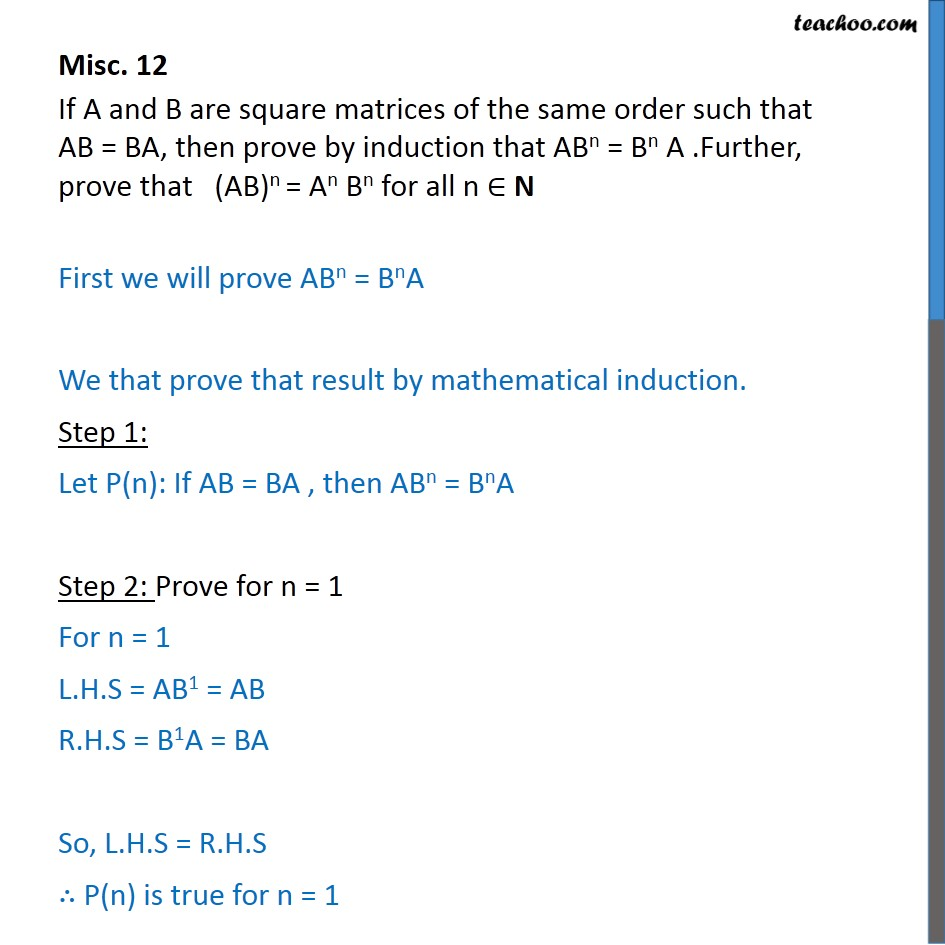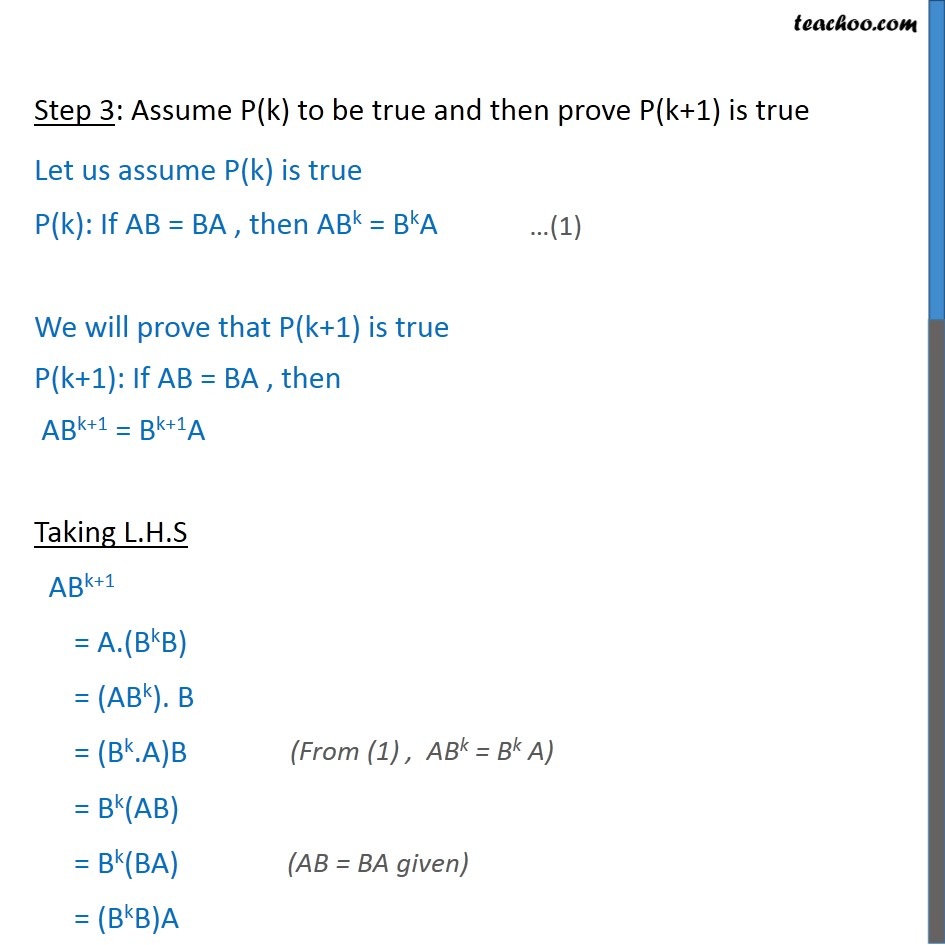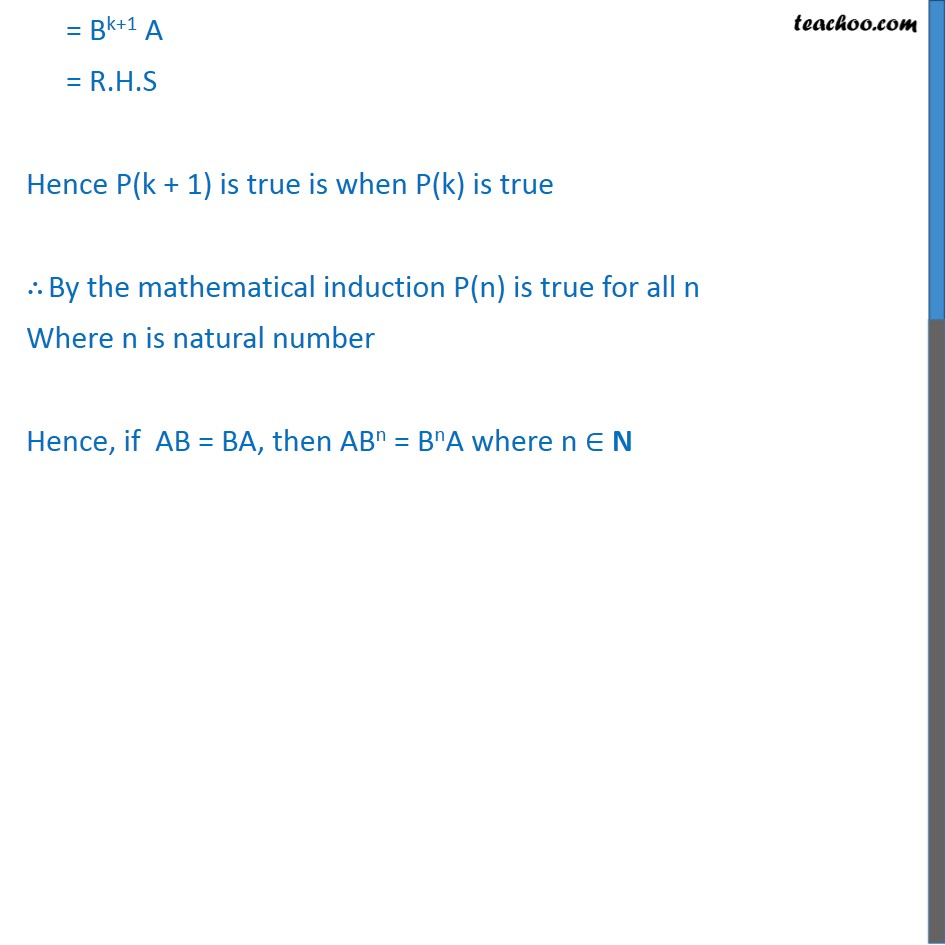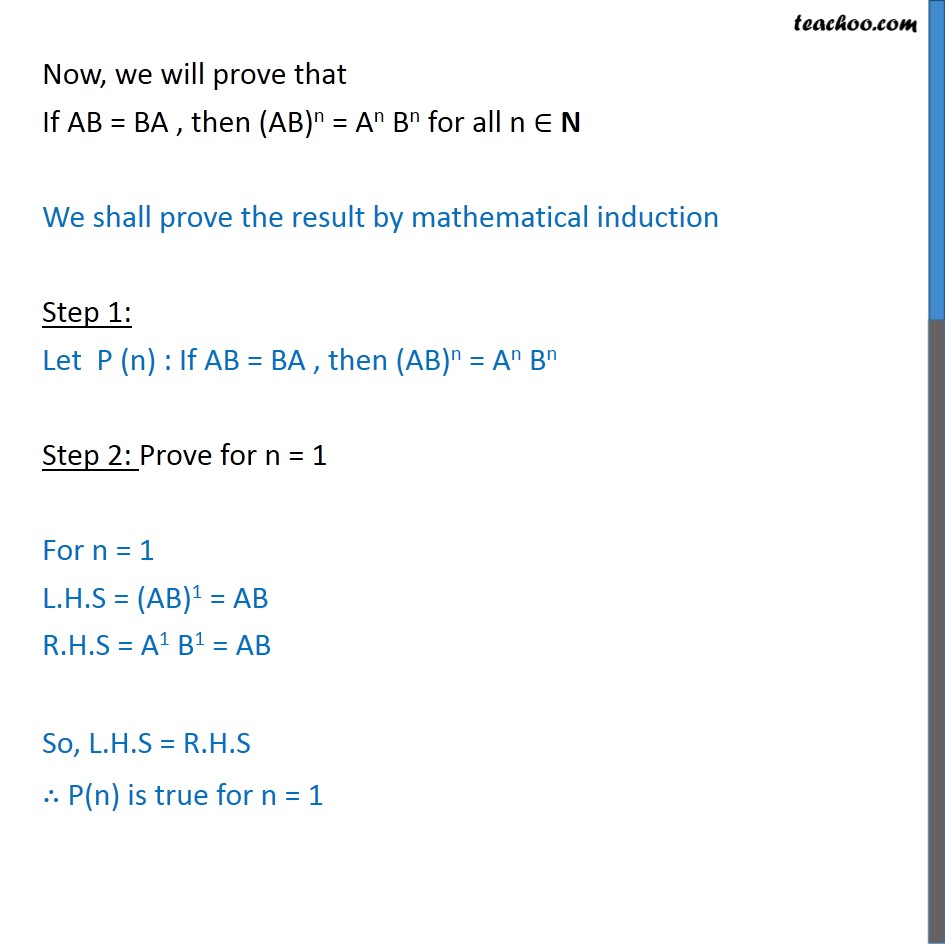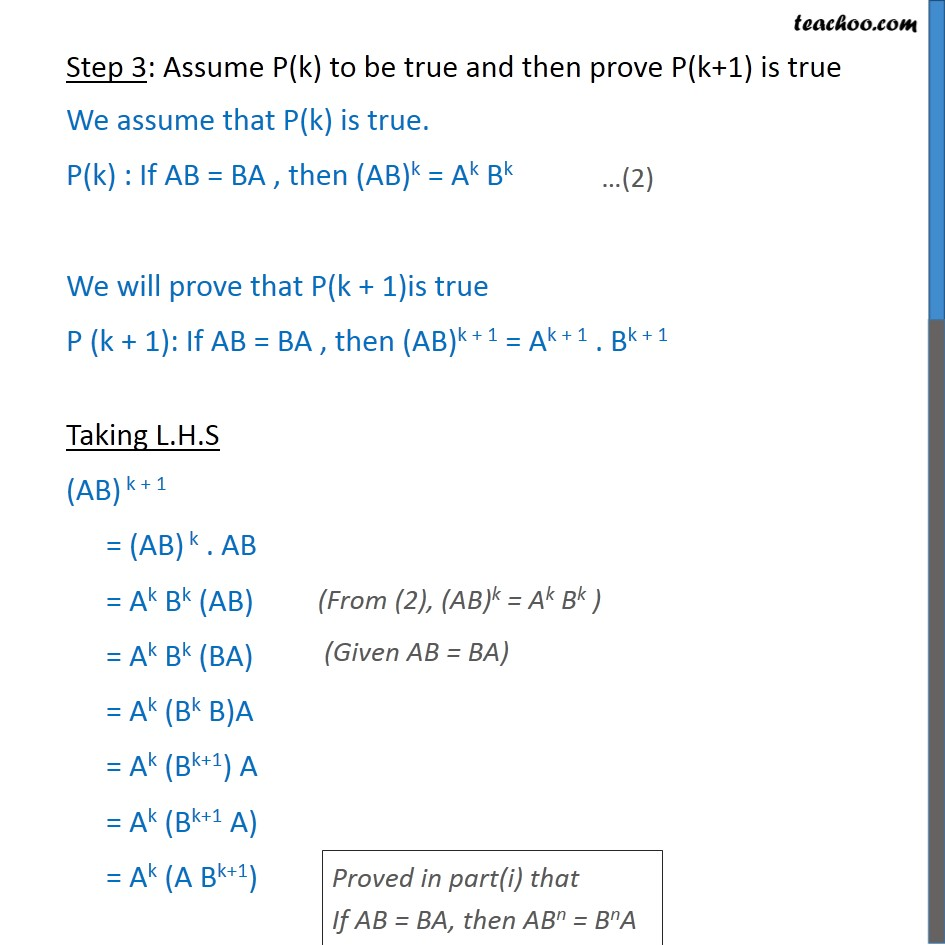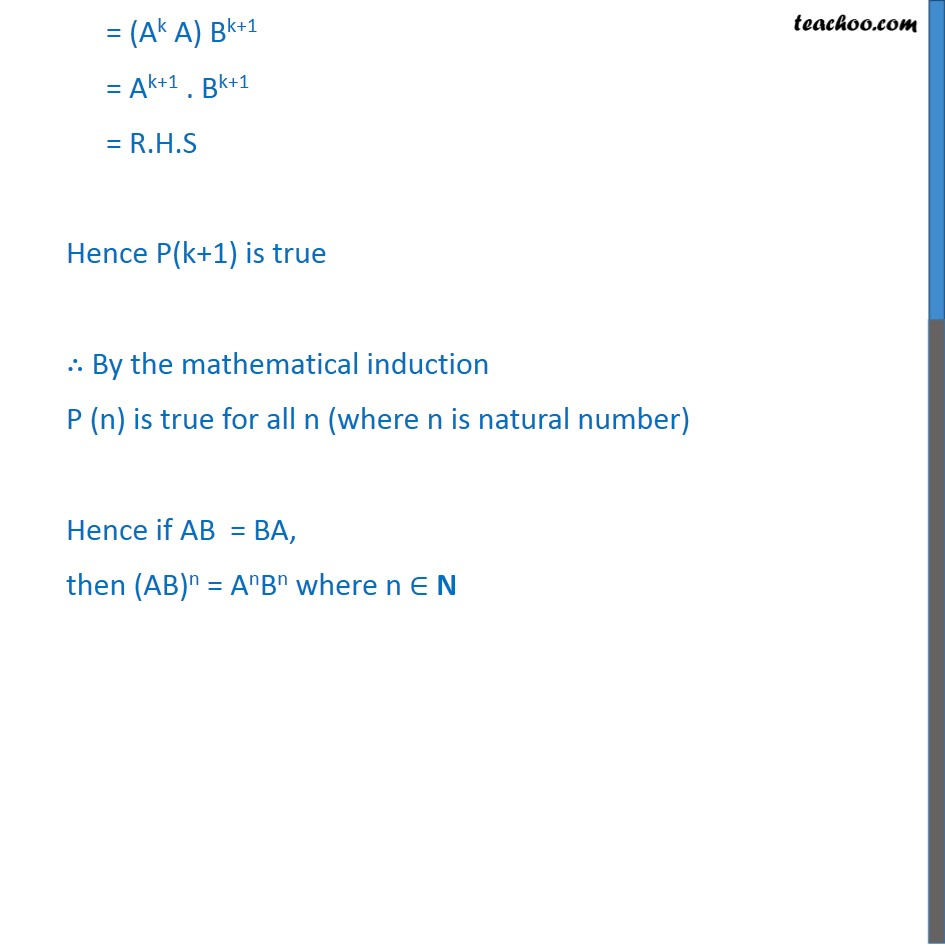Learn in your speed, with individual attention - Teachoo Maths 1-on-1 Class

### Transcript

Misc. 12 If A and B are square matrices of the same order such that AB = BA, then prove by induction that ABn = Bn A .Further, prove that (AB)n = An Bn for all n ∈ N First we will prove ABn = BnA We that prove that result by mathematical induction. Step 1: Let P(n): If AB = BA , then ABn = BnA Step 2: Prove for n = 1 For n = 1 L.H.S = AB1 = AB R.H.S = B1A = BA So, L.H.S = R.H.S ∴ P(n) is true for n = 1 Step 3: Assume P(k) to be true and then prove P(k+1) is true Let us assume P(k) is true P(k): If AB = BA , then ABk = BkA We will prove that P(k+1) is true P(k+1): If AB = BA , then ABk+1 = Bk+1A Taking L.H.S ABk+1 = A.(BkB) = (ABk). B = (Bk.A)B = Bk(AB) = Bk(BA) = (BkB)A = Bk+1 A = R.H.S Hence P(k + 1) is true is when P(k) is true ∴ By the mathematical induction P(n) is true for all n Where n is natural number Hence, if AB = BA, then ABn = BnA where n ∈ N Now, we will prove that If AB = BA , then (AB)n = An Bn for all n ∈ N We shall prove the result by mathematical induction Step 1: Let P (n) : If AB = BA , then (AB)n = An Bn Step 2: Prove for n = 1 For n = 1 L.H.S = (AB)1 = AB R.H.S = A1 B1 = AB So, L.H.S = R.H.S ∴ P(n) is true for n = 1 Step 3: Assume P(k) to be true and then prove P(k+1) is true We assume that P(k) is true. P(k) : If AB = BA , then (AB)k = Ak Bk We will prove that P(k + 1)is true P (k + 1): If AB = BA , then (AB)k + 1 = Ak + 1 . Bk + 1 Taking L.H.S (AB) k + 1 = (AB) k . AB = Ak Bk (AB) = Ak Bk (BA) = Ak (Bk B)A = Ak (Bk+1) A = Ak (Bk+1 A) = Ak (A Bk+1) = (Ak A) Bk+1 = Ak+1 . Bk+1 = R.H.S Hence P(k+1) is true ∴ By the mathematical induction P (n) is true for all n (where n is natural number) Hence if AB = BA, then (AB)n = AnBn where n ∈ N